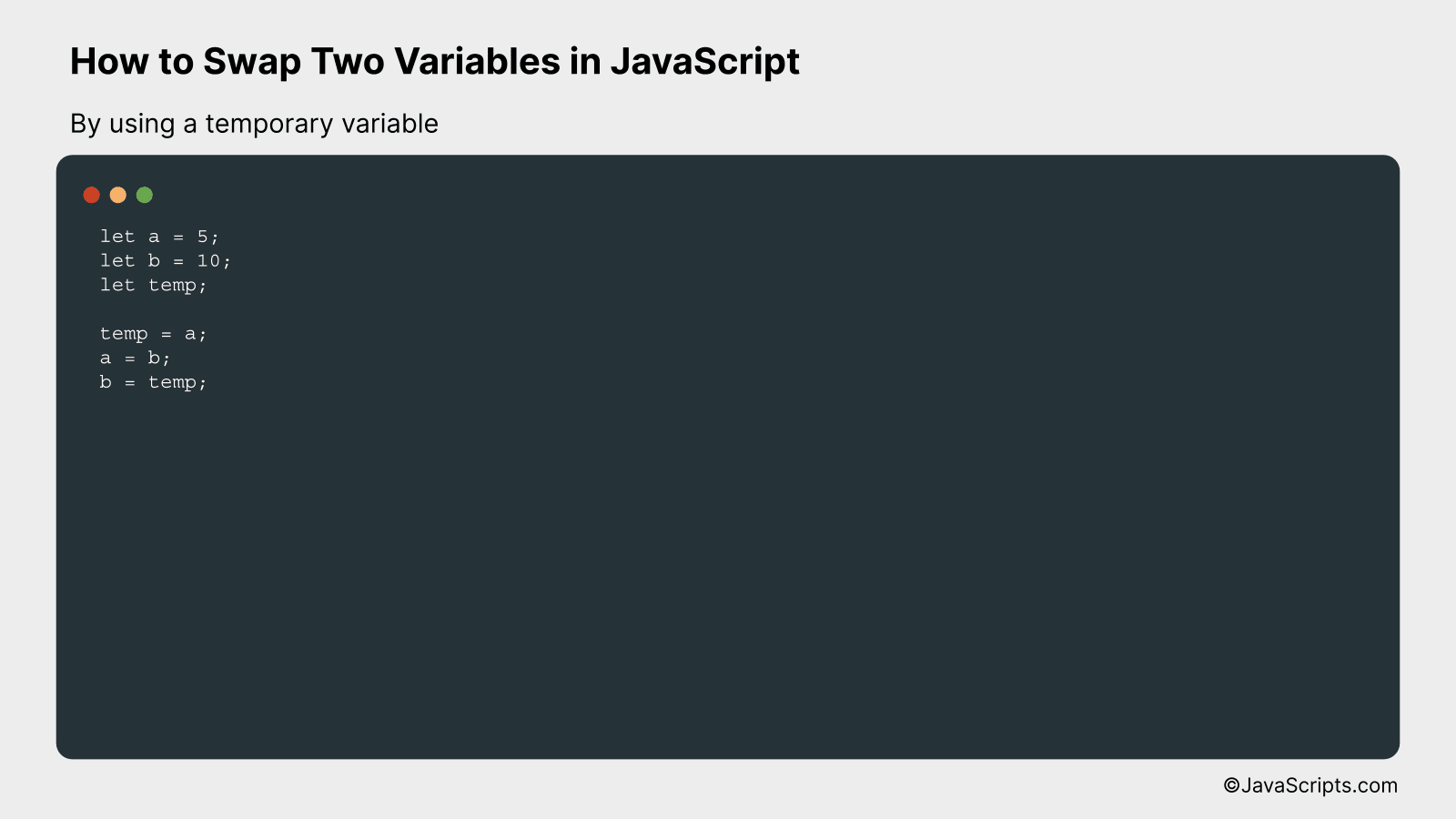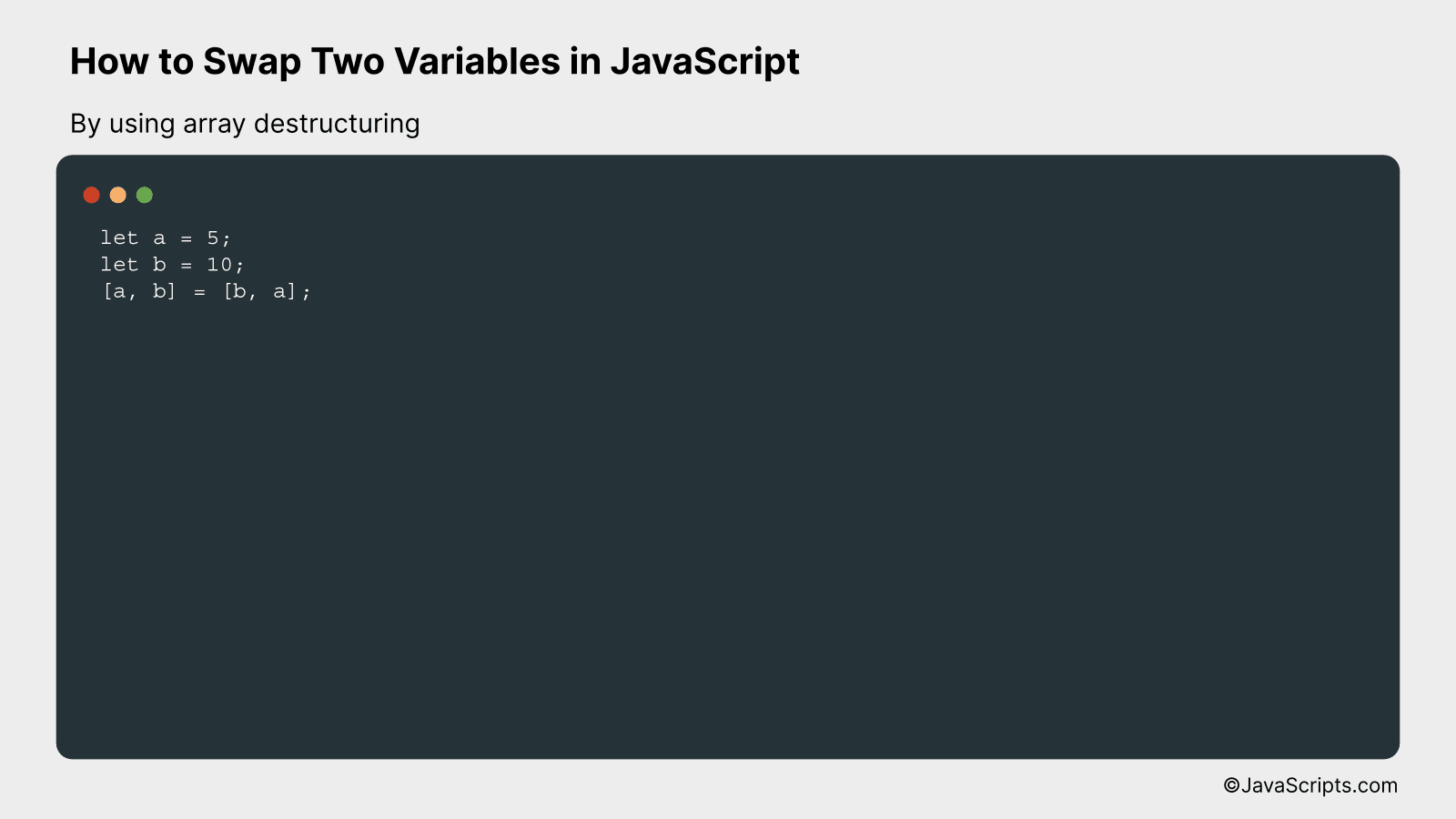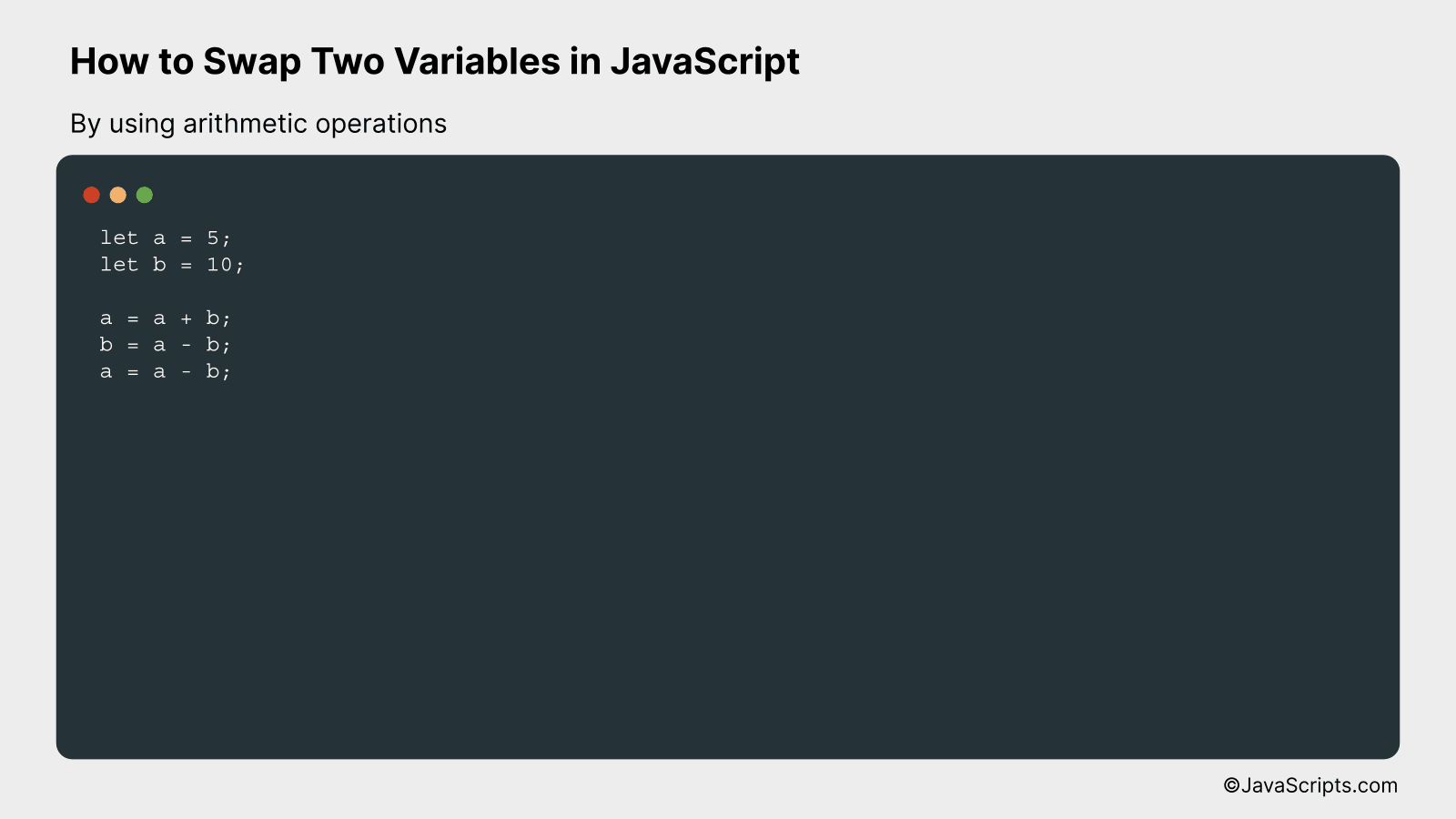# How to Swap Two Variables in JavaScript

Switching the values of two variables is a common operation in programming. Whether it’s for shuffling items in an array or reordering inputs, a good understanding of how to swap variables is a must-have skill for any coder.

Let’s decode the mystery of swapping two variables in JavaScript together. Don’t worry, whether you’re a seasoned programmer or a beginner dipping your toes into the vast ocean of JavaScript, this skill is within your grasp. It’s simpler than you might think!

In this post, we will be looking at the following 3 ways to swap two variables in JavaScript:

• By using a temporary variable
• By using array destructuring
• By using arithmetic operations

Let’s explore each…

## #1 – By using a temporary variableIn this method, we will temporarily store the value of one variable, then assign the value of the second variable to the first, and finally assign the stored value to the second variable. Here’s an example to demonstrate this:

``````
let a = 5;
let b = 10;
let temp;

temp = a;
a = b;
b = temp;
``````

### How it works

We use a temporary variable to hold the value of the first variable while we swap their values. The process is as follows:

• Create a temporary variable `temp`.
• Assign the value of variable `a` to the temporary variable `temp`.
• Assign the value of variable `b` to variable `a`.
• Finally, assign the value of the temporary variable `temp` to variable `b`.

Following these steps, the values of the two variables are successfully swapped without losing any data.

## #2 – By using array destructuringSwapping two variables in JavaScript using array destructuring involves using a one-liner syntax that easily swaps the values of the two variables without the need for a third temporary variable. Let’s take a look at an example to better understand how it works:

``````
let a = 5;
let b = 10;
[a, b] = [b, a];
``````

### How it works

In this approach, the array destructuring syntax is used to swap the values of two variables. Here’s a step-by-step explanation of how it works:

• Create two variables (`let a = 5;` and `let b = 10;`) that need to be swapped.
• Use the array destructuring syntax (`[a, b] = [b, a];`), which creates a temporary array with the values of `b` and `a`.
• Assign the values of the temporary array to variables `a` and `b` in the order they appear in the array, effectively swapping their values.

By using this technique, you can swap the values of two variables without the need for an additional temporary variable.

## #3 – By using arithmetic operationsSwapping two variables in JavaScript using arithmetic operations involves adding and subtracting the values of the variables to exchange their values without using a temporary variable. Let’s illustrate this with an example:

``````
let a = 5;
let b = 10;

a = a + b;
b = a - b;
a = a - b;
``````

### How it works

This method uses the addition and subtraction operations to swap the values of two variables, without the need for a temporary variable. Here’s a step-by-step explanation:

• Step 1: Add the values of ‘a’ and ‘b’ and store the result in ‘a’. Now ‘a’ contains the sum of both values (a = a + b).
• Step 2: Subtract the original value of ‘b’ from the new value of ‘a’ (which is the sum of both values) and store the result in ‘b’. Now ‘b’ contains the original value of ‘a’ (b = a – b).
• Step 3: Subtract the value of ‘b’ (which is the original value of ‘a’) from the value of ‘a’ (which is the sum of both values) and store the result in ‘a’. Now ‘a’ contains the original value of ‘b’ (a = a – b).

At the end of these steps, the values of ‘a’ and ‘b’ have been successfully swapped.

Related:

In conclusion, swapping two variables in JavaScript is a straightforward process. Through the use of a temporary variable or the ES6 destructuring assignment, it can be done effortlessly and efficiently.

Remember, a clear understanding of these methods helps in writing cleaner and more efficient code. So, practice using both approaches and see which one works the best for you.